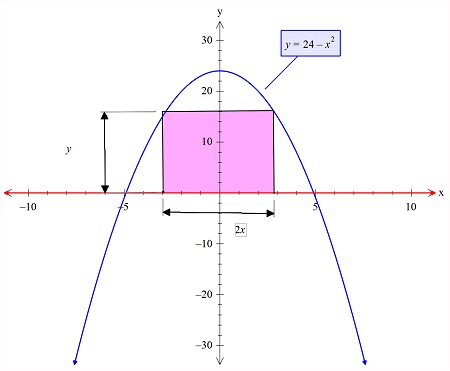# What are the dimensions of the largest rectangle that can be inscribed in a region bounded by the...

## Question:

What are the dimensions of the largest rectangle that can be inscribed in a region bounded by the graph {eq}y=24-x^2 {/eq} and the x-axis?

## Finding Minima & Maxima:

The given problem is the fantastic example of the maxima and minima concept in calculus. So here we will apply the concept of differentiation and the area of the geometric shape formulas.

In the figure below we have plotted the rectangle and the two curves, as shown:Not the get the largest rectangle we need to have the maximum area rectangle shown in pink. So we will maximize the area of this rectangle.

Now the area of the rectangle is:

{eq}A= 2xy\\ =2x(24-x^2)\\ {/eq}

Now the maximization is done by differentiation method, as follows:

{eq}A'=0\\ \Rightarrow \frac{d}{dx}\left(2x\left(24-x^2\right)\right)=0\\ \Rightarrow 2\left(\frac{d}{dx}\left(x\right)\left(24-x^2\right)+\frac{d}{dx}\left(24-x^2\right)x\right)=0\\ \Rightarrow 2\left(24-3x^2\right)=0\\ \Rightarrow x=2\sqrt{2} {/eq}

So the dimension of the largest rectangle is:

{eq}length=2x=4\sqrt{2}\\ width=y=24-(2\sqrt{2})^2=16 {/eq}